What is cannizaro reaction? Explain with example.

Aldehydes which do not have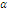-hydrogen atom, such as formaldehyte and benzaldehyte, when heated with concentrated (50%)alkali solution, give a mixture of alcohol and the salt of a carboxylic acid.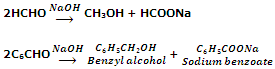In this reaction, the aldehyde undergoes disproportionate. It implies that one molecule of aldehydes is oxidized to carboxylic acid and the other is reduced to alcohol. Ketones do not provide this reaction.
The reaction is also possible in all compounds which lack-hydrogen atom. For example,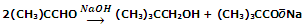It must be noted that in crossed Cannizzaro's reaction, when an aldehydes is treated with formaldehyde and aqueous basic solution then it is formaldehyde that undergoes oxidation.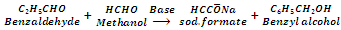Compounds containing two carbonyl groups undergo internal Cannizzaro's reaction.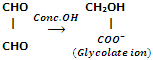On the other hand, aldehydes containing-hydrogen atoms when heated with concentrated alkali give brown resinous mass.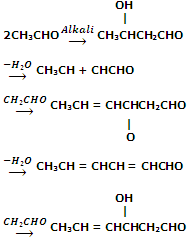#### Related Questions in Chemistry

• ##### Q :Problem on distribution law The

The distribution law is exerted for the distribution of basic acid among: (i) Water and ethyl alcohol (ii) Water and amyl alcohol (iii) Water and sulphuric acid (iv) Water and liquor ammonia What is the right answer.

• ##### Q :What do you mean by the term hydra What

What do you mean by the term hydra? Briefly define it.

• ##### Q :Number of moles of potassium chloride

Choose the right answer from following. The number of moles of KCL in 1000ml of 3 molar solution is: (a)1 (b)2 (c)3 (d)1.5

• ##### Q :Unit of molality Select the right

Select the right answer of the question. The unit of molality is: (a) Mole per litre (b) Mole per kilogram (c) Per mole per litre (d) Mole litre

• ##### Q :Problem on equilibrium constant Ethanol

Ethanol is manufactured from carbon monoxide and hydrogen at 600 K and 20 bars according to the reaction2 C0(g) + 4 H2(g) ↔ C2H5OH(g) + H2O (g)The feed stream contains 60 mol% H2, 20 m

• ##### Q :Distribution law Help me to go through

Help me to go through this problem. The distribution law is applied for the distribution of basic acid between : (a) Water and ethyl alcohol (b) Water and amyl alcohol (c) Water and sulphuric acid (d) Water and liquor ammonia

• ##### Q :Neutralization of sodium hydroxide How

How much of NaOH is needed to neutralise 1500 cm3 of 0.1N HCl (given = At. wt. of Na =23): (i) 4 g  (ii) 6 g (iii) 40 g  (iv) 60 g

• ##### Q :Moles of chloride ion Select the right

Select the right answer of the question. A solution of CaCl2 is 0.5 mol litre , then the moles of chloride ion in 500ml will be : (a) 0.25 (b) 0.50 (c) 0.75 (d)1.00

• ##### Q :Finding Normality Can someone please

Can someone please help me in getting through this problem. Concentrated H2SO4 has a density of 1.98 gm/ml and is 98% H2SO4 by weight. The normality is: (a) 2 N  (b) 19.8 N  (c) 39.6 N  (d) 98

• ##### Q :Calculating molarity of a solution

Select the right answer of the question .The molarity of a 0.2 N N2Co3 solution will be: (a) 0.05 M (b) 0.2 M (c) 0.1 M (d)0.4 M